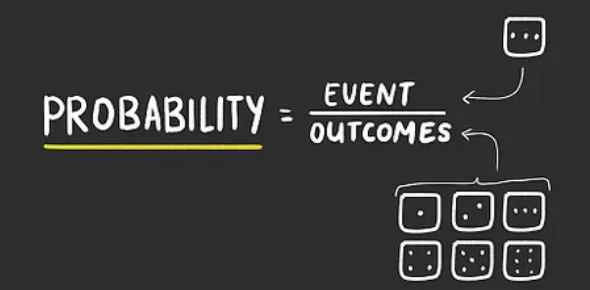# Probability Quiz: Math Trivia Test!

10 Questions | Total Attempts: 68SettingsProbability is the likelihood of an event occurring; One way someone can figure out the likelihood of events happening is by checking the total number of outcomes versus the outcome intended. In this special quiz, you will be expected to test your understanding of different probabilities and different results in cases provided. Have a try and see how much practice you may need.

• 1.
A drawer has 10 socks in it: 3 red, 2 blue, and 5 black. 2 socks are randomly selected, one at a time. What is the probability that the first sock is red?
• 2.
A drawer has 10 socks in it: 3 red, 2 blue, and 5 black. 2 socks are randomly selected, one at a time. ​What is the probability that the second sock is also red?
• 3.
A drawer has 10 socks in it: 3 red, 2 blue, and 5 black. ​What is the probability that the first 2 socks randomly selected will both be red, if only one sock is drawn out at a time?
• 4.
A class contains 20 females (12 have above 80% and 8 are below 80%) and 10 males (5 are above 80% and 5 are below 80%). If one student is randomly selected, what is the probability that that person is male?
• 5.
A class contains 20 females (12 have above 80% and 8 are below 80%) and 10 males (5 are above 80% and 5 are below 80%). If one student is randomly selected, what is the probability that the person has over 80%?
• 6.
A class contains 20 females (12 have above 80% and 8 are below 80%) and 10 males (5 are above 80% and 5 are below 80%). If one student is randomly selected, what is the probability that it will be a male or a person who has over 80% in the course?
• 7.
A game consists of
• tossing a coin,
• drawing a card from a standard deck
• and rolling a single die.
What is the probability that you flip heads?
• 8.
A game consists of
• tossing a coin,
• drawing a card from a standard deck
• and rolling a single die.
What is the probability that you draw an ace?
• 9.
A game consists of
• tossing a coin,
• drawing a card from a standard deck
• and rolling a single die.
What is the probability that you roll an even number?
• 10.
A game consists of
• tossing a coin,
• drawing a card from a standard deck
• and rolling a single die.
What is the probability that you flip heads, draw an ace, and roll an even number?
Related TopicsBack to top